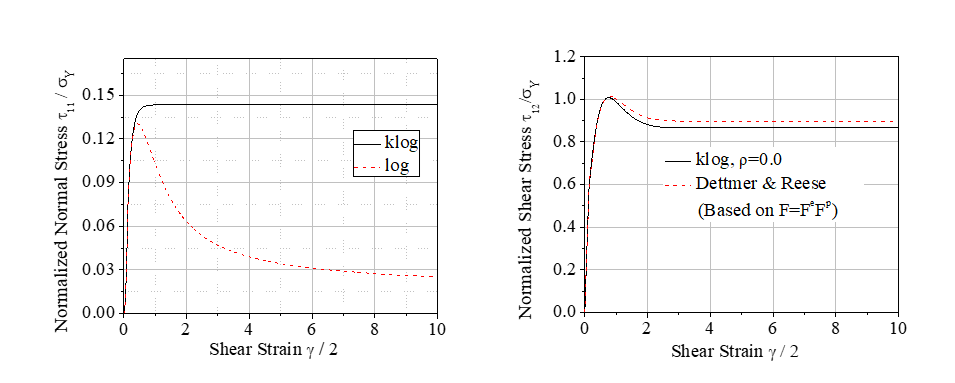# Is an additive decomposition of a rate of deformation and objective stress rates passé?

A half a century old idea of decomposing the rate of deformation into elastic and plastic parts is critically examined. We show that even the most recent additive variant based on the logarithmic rate is inconsistent with the notion of elasticity in so-called unloading stress ratchetting obstacle test while the earlier corotational variants based on the Jaumann and Green-Naghdi rates are well known not to be integrable. We then develop a stress-dependent so-called kinetic logarithmic stress rate that is both integrable and passes the unloading stress ratchetting obstacle test. We also demonstrate that the additive hypo-elasto-plasticity model based on the proposed kinetic logarithmic rate is weakly invariant under isochoric reference change for simple materials in the sense of Noll. Comparison to classical logarithmic rate and multiplicative decomposition of deformation gradient in shear test problem are shown below.(a) (b)

Simple shear test. Comparison of kinetic logarithmic rate (klog) with (a) classical logarithmic strain (log) for normal stress in elastic-perfectly plastic material and (b) multiplicative decomposition for shear stress in Armstrong–Frederick kinematic hardening material